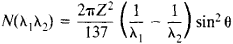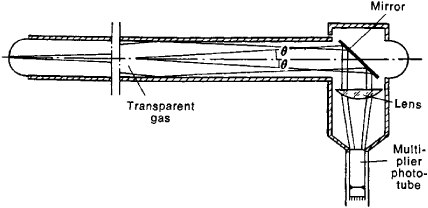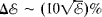# Cherenkov Counter

The following article is from The Great Soviet Encyclopedia (1979). It might be outdated or ideologically biased.

## Cherenkov Counter

(or Čerenkov counter), a device that uses Cherenkov radiation to detect charged particles or gamma rays.

If a charged particle moves in a medium at a velocity v that is greater than the phase velocity of light waves in the medium, the particle emits Cherenkov radiation. The phase velocity of light waves in a given medium is equal to c/n, where n is the refractive index of the medium and c is the speed of light in vacuum. Cherenkov radiation is emitted in a specific direction, and the angle θ between the direction of the radiation and the trajectory of the particle is related to v and n. The relationship is given by the equation cos θ; = c/vn = 1/βn, where β = v/c. The yield N of Cherenkov radiation per centimeter of path between wavelength limits λ1 and λ2 is specified by the equationwhere Z is the charge of the particle in units of the charge of the electron.

In contrast to a scintillation counter, in which particles with any velocity are detected and the radiation is both isotropic and delayed, the light in a Cherenkov counter is emitted only by particles whose velocities vc/n, that is, by particles for which β ≥ 1/n. The emission occurs at the same time as the passage of the particles and at an angle θ to the trajectory of the particles. The angle θ; and the radiation yield increase with increasing particle velocity above a certain threshold velocity. For particle velocities that approach the speed of light—that is, for (1 – β) << 1—the angle attains a maximum value θmax = cos–1 (1/n). As a rule, the amount of light emitted in a Cherenkov counter is a few percent of a light pulse in a scintillation counter.

The basic components of a Cherenkov counter are a transparent substance in which v > c/n; an optical system, which focuses the light; and one or more multiplier phototubes, which convert a light pulse into an electrical signal (see Figure 1). The transparent substance may be a solid, a liquid, or a gas. It should be transparent to Cherenkov radiation and have a low level of scintillation, since scintillations produce background signals. Among the conventional substances used are organic glass (n = 1.5), lead glass (n = 1.5), and water (n = 1.33).Figure 1. Schematic of a threshold gas Cherenkov counter used with the 70-GeV particle accelerator of the Institute of High-energy Physics (USSR). The Cherenkov light is collected at the cathode of the multiplier phototube by means of an optical system consisting of a plane mirror and a quartz lens.

Cherenkov counters are widely used in experiments employing particle accelerators, since the counters make it possible to pick out particles whose velocity lies in a specific range. As accelerator energies and, consequently, particle energies have increased, gas Cherenkov counters have been especially widely used. Gas Cherenkov counters can single out ultrarelativistic particles, for which (1 – β) << 1. The angle θ; at which the radiation is emitted is very small in the case of a gas, and the radiation yield per unit path is low. To obtain a flash of light that is sufficient for the detection of a particle, the length of a gas Cherenkov counter must be increased to 10 m or longer. In gas Cherenkov counters, the refractive index may be varied continuously within a given range by changing the operating gas pressure.

Three types of Cherenkov counters are distinguished: threshold, differential, and total-absorption. The main characteristics of threshold and differential Cherenkov counters are the detection efficiency and the velocity resolution; the latter is a measure of a counter’s ability to distinguish two particles moving with nearly the same velocity. A threshold Cherenkov counter should detect all particles with velocities greater than some threshold value. Therefore, a threshold counter’s optical system, which is a combination of lenses and mirrors, should—if possible—collect all the emitted light at the cathode of the multiplier phototube.

Differential Cherenkov counters detect particles whose velocity lies between certain limits v1 and v2. In conventional differential Cherenkov counters, the desired particles are detected by using the optical system to select the light that is emitted at an angle lying between the corresponding limits θ1, and θ2. A lens or a spherical mirror placed in the path of the Cherenkov light focuses the light that is emitted at an angle θ into a ring of radius R = fθ where f is the focal length of the lens or mirror. If an annular slit diaphragm is placed at the focus of the optical system and one or more multiplier phototubes are placed behind the diaphragm, light will be detected only for particles that radiate at an angle lying within certain limits. A differential Cherenkov counter with a precision optical system makes it possible to single out particles whose velocity differs by just 10–6 from the velocity of other particles. Such Cherenkov counters require special control over the gas pressure and the formation of a parallel particle beam.

Total-absorption Cherenkov counters are intended for the detection and spectrometry of electrons and gamma rays. In contrast to threshold and differential Cherenkov counters, in which a particle loses a negligibly small fraction of its energy in the transparent substance, a total-absorption Cherenkov counter contains a very thick slab of transparent material, in which an electron or a gamma ray produces an electron-photon avalanche and loses all or most of its energy. As a rule, the slab is made of glass with a high lead content. An electron with an energy of up to 10 giga-electron volts (GeV) may be virtually completely stopped in a slab of such glass with a thickness of, for example, 40 cm. The amount of light that is emitted in a total-absorption Cherenkov counter is proportional to the energy of the initial electron or gamma ray. The energy resolution Δℰ of a total-absorption Cherenkov counter is energy dependent and, for the most sensitive multiplier phototubes, may be specified by the equation, where ℰ is the electron energy in gigaelectron volts.

### REFERENCES

Jelley, J. Cherenkovskoe izluchenie i ego primeneniia. Moscow, 1960. (Translated from English.)
Zrelov, V. P. Izluchenie Vavilova-Cherenkova i ego primenenie v fizike vysokikh energii, parts 1–2. Moscow, 1968.

V. S. KAFTANOV